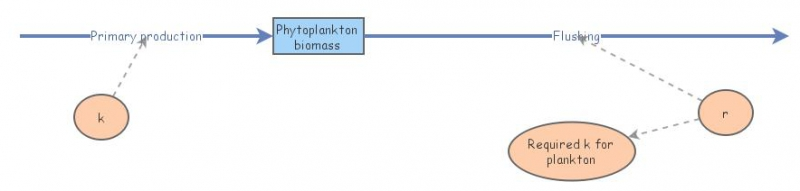Phytoplankton blooms in estuariesThis model implements the equations proposed by Ketchum in 1954. The rationale behind the concept is that only phytoplankton that grows above a certain rate will not be flushed out of an estuary.

For biological processes:

Pt  =  Po exp(kt)

Where Pt is the phytoplankton biomass at time t, Po is the initial biomass, and k is the growth rate.

For physical processes:

Pm  =  Po (1-r)^m

Where Pm is the phytoplankton biomass after m tidal cycles, and r is the exchange ratio (proportion of estuary water which does not return each tidal cycle).

By substitution, and replacing t by m in the first equation, we get:

Pm = Poexp(km).(1-r)^m

For phytoplankton to exist in an estuary, Pm = Po (at least), i.e. 1 / (1-r)^m = exp(km)
ln(1) - m.ln(1-r) = km
-m.ln(1-r) = km
k = -ln(1-r)

Ketchum (1954) Relation between circulation and planktonic populations in estuaries. Ecology 35: 191-200.

In 2005, Ferreira and co-workers showed that this balance has direct implications on biodiversity of estuarine phytoplankton, and discussed how this could be relevant for water management, in particular for the EU Water Framework Directive 60/2000/EC (Ecological Modelling, 187(4) 513-523).